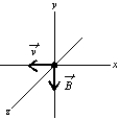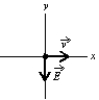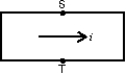# Fundamentals of Physics Study Set 2

Physics & Astronomy

## Quiz 28 :Magnetic FieldsLooking for Introductory Physics Homework Help?

## Quiz 28 :Magnetic Fields

Showing 1 - 20 of 51Units of a magnetic field might be:
Free
Multiple Choice

DThe direction of the magnetic field in a certain region of space is determined by firing a test charge into the region with its velocity in various directions in different trials.The field direction is:
Free
Multiple Choice

AAn electron is moving north in a region where the magnetic field is south.The magnetic force exerted on the electron is:
Free
Multiple Choice

AA magnetic field exerts a force on a charged particle:
Multiple ChoiceAn electron travels due north through a vacuum in a region of uniform magnetic field that is also directed due north.It will:
Multiple ChoiceAn electron and a proton are both initially moving with the same speed and in the same direction at 90˚ to the same uniform magnetic field.They experience magnetic forces, which are initially:
Multiple ChoiceA hydrogen atom that has lost its electron is moving east in a region where the magnetic field is directed from south to north.It will be deflected:
Multiple ChoiceA beam of electrons is sent horizontally down the axis of a tube to strike a fluorescent screen at the end of the tube.On the way, the electrons encounter a magnetic field directed vertically downward.The spot on the screen will therefore be deflected:
Multiple ChoiceAn electron moves in the negative x direction, through a uniform magnetic field that is in the negative y direction.The magnetic force on the electron is:Multiple ChoiceIn the formula F ⃗=qv ⃗×B ⃗ :
Multiple ChoiceThe magnetic force on a charged particle is in the direction of its velocity if:
Multiple ChoiceA static magnetic field CANNOT:
Multiple ChoiceAt any point the magnetic field lines are in the direction of:
Multiple ChoiceJ.J.Thomson's experiment, involving the motion of an electron beam in mutually perpendicular and fields, gave the value of:
Multiple ChoiceA charged particle is projected into a region of uniform, parallel, and fields.The force on the particle is:
Multiple ChoiceAn electron enters a region of uniform perpendicular and fields.It is observed that the velocity of the electron is unaffected.A possible explanation is:
Multiple ChoiceA uniform magnetic field is in the positive z direction.A positively charged particle is moving in the positive x direction through the field.The net force on the particle can be made zero by applying an electric field in what direction?
Multiple ChoiceAn electron is travelling in the positive x direction.A uniform electric field is in the negative y direction.If a uniform magnetic field with the appropriate magnitude and direction also exists in the region, the total force on the electron will be zero.The appropriate direction for the magnetic field is:Multiple ChoiceThe current is from left to right in the conductor shown.The magnetic field is into the page and point S is at a higher potential than point T.The charge carriers are: